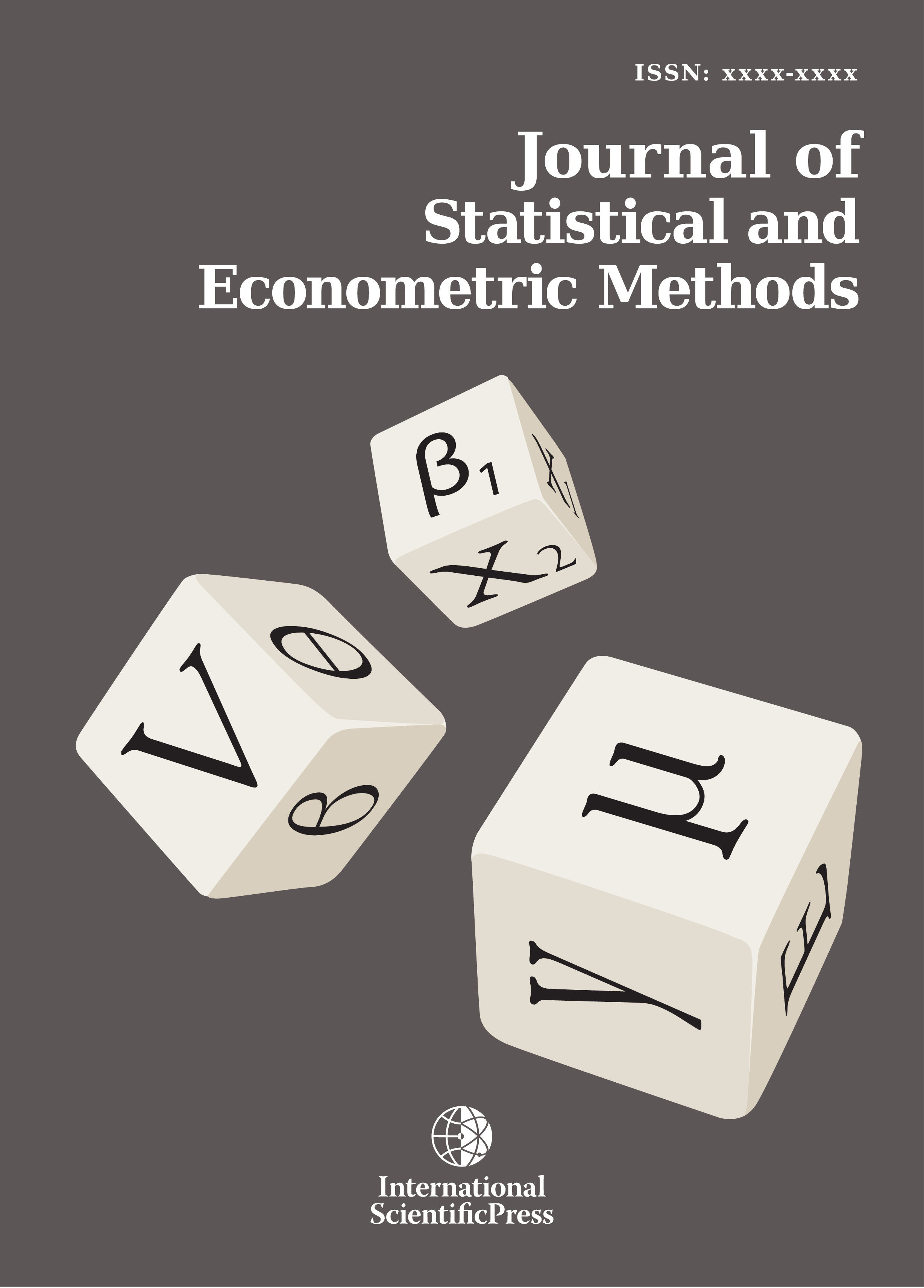# Journal of Statistical and Econometric Methods

#### Comparison of the Powers of the Kolmogorov-Smirnov Two-Sample Test and the Mann-Whitney Test for Different Kurtosis and Skewness Coefficients Using the Monte Carlo Simulation Method

•[ Download ]
• Times downloaded: 1708
• Abstract

This study aims to compare the statistical powers of the Kolmogorov-Smirnov two-sample test and the Mann-Whitney test using the Monte Carlo simulation method, for specific sample sizes. The simulation results showed that the Mann-Whitney Test was more powerful for (5, 10) and (10, 5) sample sizes when the standard deviation rates were 2 and 1/2; for the (5, 20) sample size when the standard deviation rates were 2, 3, and 1/2; and for the (20, 5) sample size when the standard deviation rates were 1/2, 1/3, and 1/4. The Kolmogorov-Smirnov two-sample test was more powerful for (5, 10) and (10, 5) sample sizes when standard deviation rates were 3, 4, and 1/4; for the (10, 20) sample size for all standard deviation rates; for the (20, 10) sample size excepting the standard deviation of 1/2; and for the (20, 5) sample size when standard deviations were 2, 3, and 4.ISSN: 2241-0376 (Online)
2241-0384 (Print)